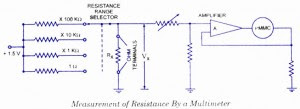## Audio

[Audio][slideshow]

### Electronic Multimeters

It is one of the most versatile general purpose instruments capable of measuring dc and ac voltages as well as current and resistances. The solid-state electronic multimeter (or VOM) generally consists of the following elements.1. A balanced bridge dc am­plifier and a PMMC meter.
2. An attenuator in input stage to select the proper voltage range.

3. A rectifier for convert­ing of an ac input volt­age to proportionate dc value.

4. An internal battery and additional circuitry for providing the capability of resistance measure­ment.

5. A function switchfor selecting various meas­urement functions of the meter such as voltage, current or resistance.

In addition, the instrument is usually provided with a built-in power supply for operation on ac mains and, in most cases, one or more batteries for operation as a portable test instrument.

The schematic diagram of a balanced-bridge dc amplifier using two field effect transistors (FETs) is given in figure. It is to be noted that two FETs used in circuit should be reasonably well matched for current gain to ensure circuit thermal stability. The two FETs and the source resistors Rx and R2, together with zero adjust control resistor R3, constitute a bridge circuit. The PMMC meter is connected between the source terminals of the FETs, representing two opposite corners of the bridge.

In the absence of input signal, the gate terminals of the FETs are at ground potential and the transistors operate under identical quiescent conditions. Ideally no current should flow through the PMMC movement but in practice, on account of some mismatch between the two FETs and slight tolerance differences in the values of various resistors a current does flow and causes the meter movement to deflect from zero position. This current is reduced zero by the adjust control resistor R3. Now the bridge is balanced. With a positive input signal applied to the gate of input transistor T1 its drain current creases causing the voltage at the source terminal to rise. The resulting unbalance between the two transistors T1 and T2 source voltages is shown by the meter movement, whose scale is calibrated in terms of the magnitude of the applied input voltage. The maximum voltage that can be applied to the gate of input transistor T1 is deter­mined by its operating range, which is usually of the order of a few volts. The range of the bridge can easily be extended by employing an input attenuator or a range switch, as illustrated in figure.

The unknown dc input voltage is applied through a large resistor in the probe body to a resistive voltage divider. Thus, with the range switch in the 3-V position as illustrated, the voltage at the gate of the input transistor T1is developed across 8 MQ resistor of the total resistance of 11.3 MQ and the circuit is so arranged that the PMMC meter gives full scale deflection with 3 V applied to the tip of the probe. With the range switch in 1,200 V position, the gate voltage is developed across 20 kilo ohm of the total divider resistance of 11.3 Mega ohms and an input voltage of 1,200 V will he required to cause the full-scale meter deflection.

### Measurement of Resistance by Multimeter or VOM.Measurement of resistance by a multimeter

When the multimeter’s func­tion switch is placed in the OHM position, the unknown resistor is connected in series with an internal battery, and the PMMC meter simply measure the voltage drop across it (unknown resistor, Rx).

A typical circuit is given in figure. In the given circuit, a separate divider network, employed only for the meas­urement of resist­ance, provides a number of different resistance ranges. With the unknown resistor Rx con­nected to the OHM terminals of the multimeter, the battery supplies current through one of the range resistors and the unknown resistor to ground. Voltage drop across unknown resistor Rx, Vx is applied to the input of the bridge amplifier and the PMMC movement deflects. Since the voltage drop across Rx is directly proportional to its resistance, the meter scale can be calibrated in terms of Kilo ohm.

The worth noting point is that this instrument indicates increasing resistance from left to right whereas the conventional meter, indicates increasing resistance from right to left. This is because the electronic multimeter reads a large resistance as a higher voltage, whereas the conventional multimeter indicates a higher resistance as a smaller current.

[Lights][grids]